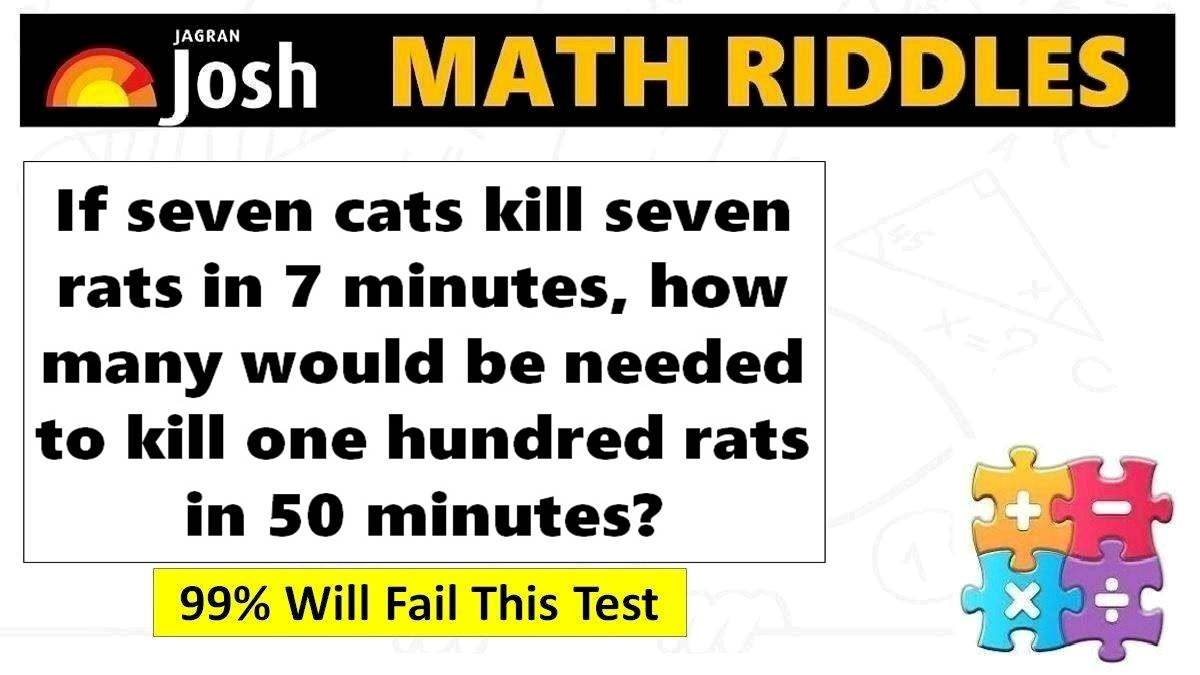# Math Riddles with Answers: 5 Easy Math Questions, Only 1% Genius Can Solve

Math Riddles Challenge: These math puzzles will trick your brain. Check your common sense level with these fun and mind bending mathematics riddles.Math Riddles with Answers: 5 Easy Math Questions, Only 1% Genius Can Solve

Math Riddles: Are you among the 1% genius who can solve these 5 math riddles in 20 seconds each? Statistics tell that 99% percent of people failed at finding the answers. These mathematics puzzles will surely a great brain exercise. You will love these math riddles, after all maths is fun, isn’t it? Let us begin.

## Solution

### Maths Logic Puzzle #1

Explanation: To kill 7 rats, it would take 7 cats: 7 × 7 = 49 mins

To kill 1 rat, it takes 1 cat, that is: 49/7 = 7 mins

Since, the job is to kill 100 rats, it would take 1 cat, that is:

7 × 100 = 700 mins

So, In order to do the job in 50 mins, we would therefore need: 700/50 = 14 cats

Therefore, it would take 14 cats 50 minutes to kill 100 rats.

### Maths Logic Puzzle #2

Explanation: Flip your phone or PC, it will be 8 + * = 16

### Maths Logic Puzzle #3

Explanation: Remove the S from Seven. It becomes Even.

### Maths Logic Puzzle #4

Explanation: As per the statement, the person finished two places ahead of the person finished last and one ahead of the person who finished 5th. Therefore, one can imply that man finished 4th and there were total of 6 contestants who were running the race.

### Maths Logic Puzzle #5

Answer: Hockey Stick costs \$49.50 and Ball costs \$0.50

## Tell us in comments: Did you solve these mathematics puzzles in less than 20 seconds each?

Check out more math puzzles!

Also Read: Math Riddles with Answers: 5 Easy Math Questions, 99% Still Fail This Test

Also Read: Math Riddles with Answers: Common Sense Test, 99% Will Fail This Test

Also Read: Math Riddles with Answers: Logic Puzzles That Will Teach Fast Maths Tricks

Get the latest General Knowledge and Current Affairs from all over India and world for all competitive exams.
खेलें हर किस्म के रोमांच से भरपूर गेम्स सिर्फ़ जागरण प्ले पर